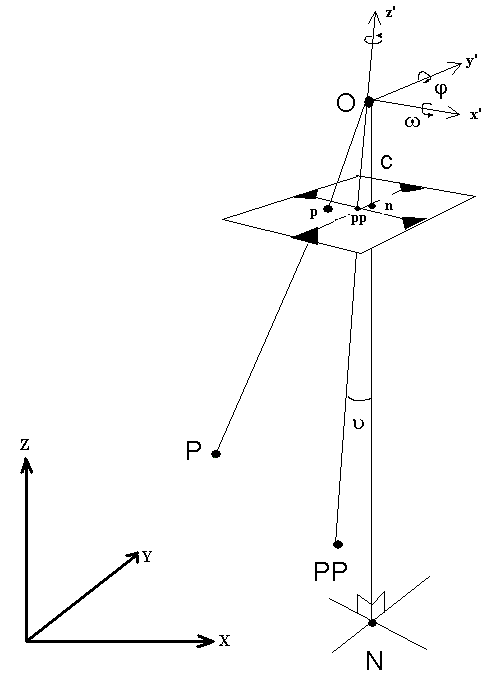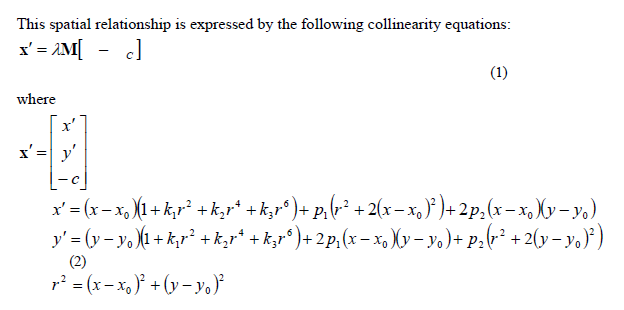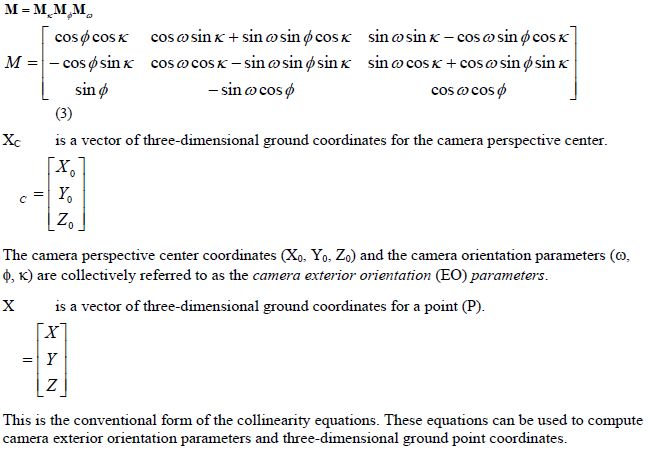# Mathematical Models for Bundle Adjustment

## Producer Field Guide

HGD_Product
Producer Field Guide
HGD_Portfolio_Suite
Producer

The following is intended to document the mathematical models required for a comprehensive bundle block adjustment, such as that provided by PhotoT. The mathematical models include standard collinearity equations, GPS antenna offset, GPS shift and drift, INS shift and drift, camera calibration, and self-calibration.

In bundle block adjustments the rays from the projection centers through the measured photo points to the object points represent a spatial pencil of rays for each photo (Figure 6).Figure 6. Image/Object Coordinate Systems of an Aerial Photographx’, y’ are image coordinates of point (p). The principal point coordinates (xo, yo), principal distance (c), radial lens distortion coefficients (k1, k2, k3), and tangential lens distortion coefficients (p1, p2) are collectively referred to as the camera interior orientation parameters.

λ is the scale per point.

M is an orthogonal rotation matrix that rotates ground-system coordinate axes parallel to corresponding image coordinate axes. M is a function of the three sequential camera orientation angles omega (ω), a rotation about the ground-system X-axis, then phi (φ), a rotation about the once-rotated Y-axis, and finally kappa (κ), a rotation about the twice-rotated Z-axis.